# 0. 目录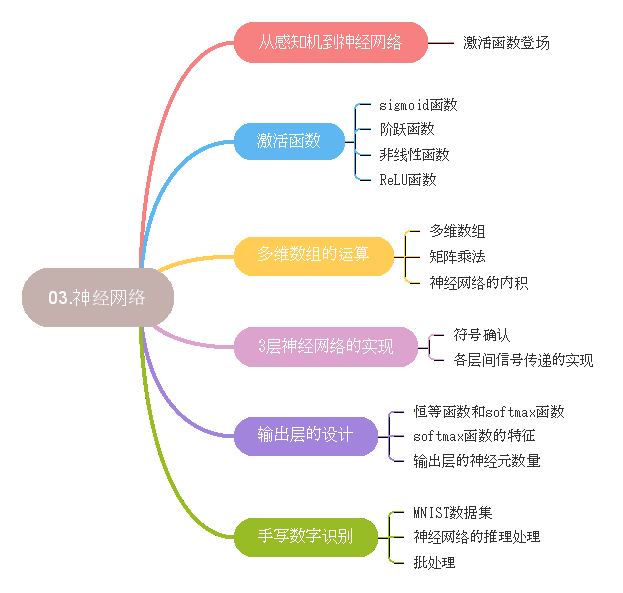# 1. 从感知机到神经网络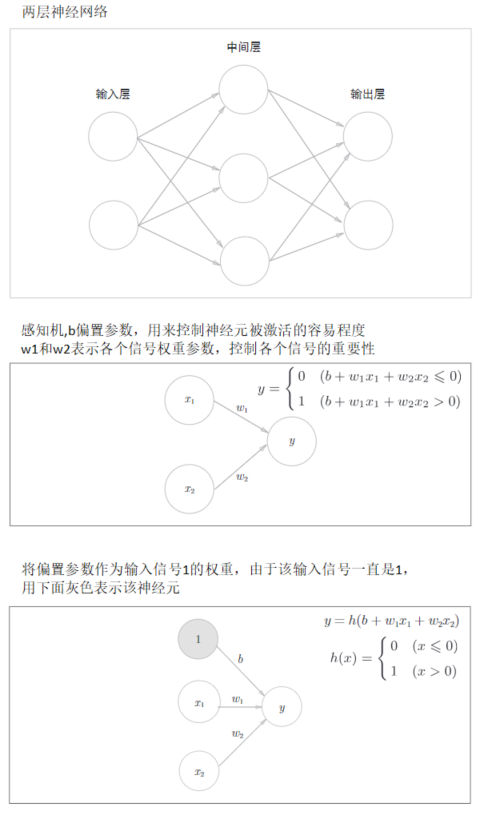# 2. 激活函数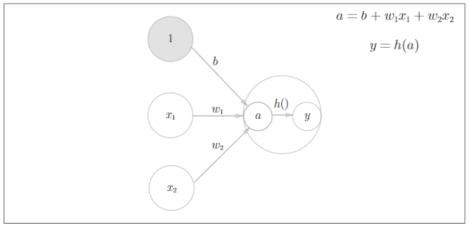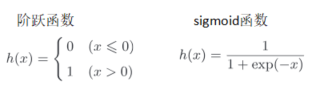## 2.1 阶跃函数

• 代码实现
``````import numpy as np

def step_function(x):
y = x > 0
print(y)
return y.astype(np.int)

x = np.array([-1,2,-2,3])
print(step_function(x))
``````

``````[False  True False  True]
[0 1 0 1]
``````
• 图形化:
``````import numpy as np
import matplotlib.pylab as plt

def step_function(x):
return np.array(x>0,dtype=np.int)

x = np.arange(-5,5,0.1)
y = step_function(x)
plt.plot(x,y)
plt.ylim(-0.1,1.1)
plt.show()
``````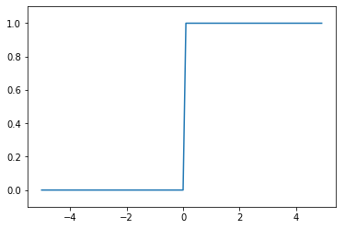## 2.2 sigmoid函数

sigmoid函数表达式参考上面，exp(-x)表示e(纳皮尔常熟,2.7182)的-x次方，神经网络中用sigmoid函数作为激活函数，进行信号转换，转换后的信号被传递给下一个神经元。

• 代码实现及图形表示
``````def sigmoid(x):
return 1 / (1 + np.exp(-x))

x = np.arange(-5,5,0.1)
y = sigmoid(x)
print("x:",x[:5],"......")
print("y:",y[:5],"......")
plt.plot(x,y)
plt.ylim(-0.1,1.1)
plt.show()
``````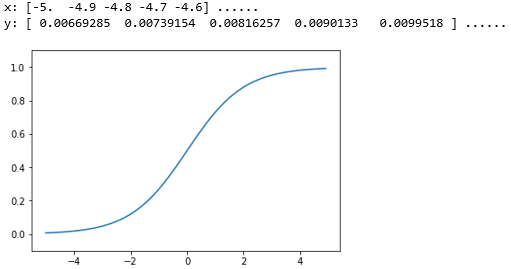## 2.3 sigmoid函数和阶跃函数比较

``````x = np.arange(-5,5,0.1)
y1 = step_function(x)
y2 = sigmoid(x)

plt.plot(x,y1)
plt.plot(x,y2)
plt.ylim(-0.1,1.1)
plt.show()
``````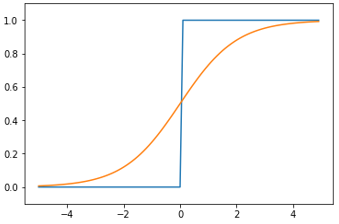• 阶跃函数只能返回0和1，而sigmoid函数返回`0.00669285`等实数，也就是说感知机中的神经元只有0和1的二元信号，而神经网络中流动的是连续的实数值信号。

• 不管输入信号多小或多大，输出信号都在0和1之间。

• 都是`非线性函数`，sigmoid函数是一条曲线，阶跃函数是一条阶梯一样的折线。

• `线性函数`: 向这个转换器输入某个值后，输出值是输入值的常数倍，如`h(x)=cx，c为常数`
• `非线性函数`: 不像线性函数那样呈现一条直线的函数

## 2.4 ReLU函数(Rectified Linear Unit)函数

ReLU函数在输入大于0时，直接输出该值，输入小于0时，输出0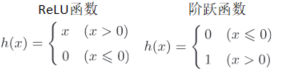``````def relu(x):
return np.maximum(0,x)

x = np.arange(-5,5,0.1)
y1 = step_function(x)
y2 = sigmoid(x)
y3 = relu(x)

plt.plot(x,y1)
plt.plot(x,y2)
plt.plot(x,y3)

plt.ylim(-0.1,5.1)
plt.show()
``````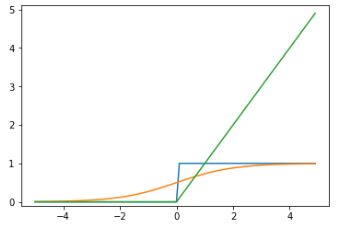# 3. 多维数组的运算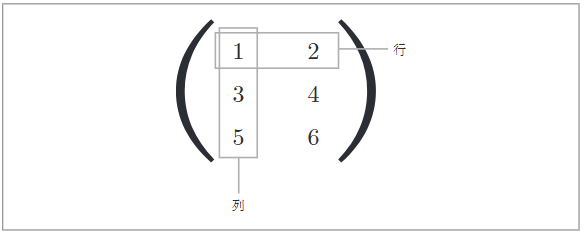``````B = np.array([[1,2],[3,4],[5,6]])
print(B)
print("形状:",B.shape)
print("行数:",B.shape)
print("列数:",B.shape)
``````
``````[[1 2]
[3 4]
[5 6]]

``````

## 3.1 矩阵乘法

1. A 的 行数，B 的 列数，决定乘积矩阵的形状。
2. A 的 列数，要与B的行数保持相同。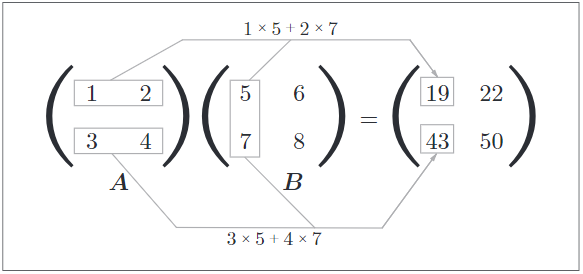• 代码 `(2, 2) * (2, 2) = (2, 2)`
``````A = np.array([[1,2],[3,4]])
B = np.array([[5,6],[7,8]])
C = np.dot(A,B)
print(C,"\n")
print("A:",A.shape,"* B:",B.shape)
print("C:",C.shape)
``````

``````[[19 22]
[43 50]]

A: (2, 2) * B: (2, 2)
C: (2, 2)
``````
• 代码 `(3, 2) * (2, 4) = (3,4)`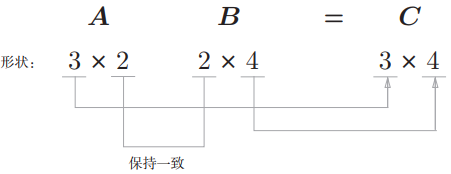``````A = np.array([[1,2],[3,4],[1,2]])
B = np.array([[5,6,5,6],[7,8,7,8]])
C = np.dot(A,B)
print(C,"\n")
print("A:",A.shape,"* B:",B.shape)
print("C:",C.shape)
``````

``````[[19 22 19 22]
[43 50 43 50]
[19 22 19 22]]

A: (3, 2) * B: (2, 4)
C: (3, 4)
``````
• 代码 `(3, 2) * (3, 4) = ERROR !`
``````A = np.array([[1,2],[3,4],[1,2]])
B = np.array([[5,6,5,6],[7,8,7,8],[7,8,7,8]])
C = np.dot(A,B)
print(C,"\n")
print("A:",A.shape,"* B:",B.shape)
print("C:",C.shape)
``````

``````ValueError: shapes (3,2) and (3,4) not aligned: 2 (dim 1) != 3 (dim 0)
``````
• 代码 `(3, 2) * (2) = (3)

A是二维矩阵，B是一维数组时，也要保持对应的维度元素个数一致。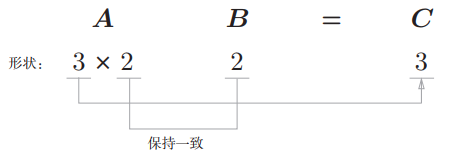``````A = np.array([[1,2],[3,4],[1,2]])
B = np.array([7,8])
C = np.dot(A,B)
print(C,"\n")
print("A:",A.shape,"* B:",B.shape)
print("C:",C.shape)
``````

``````[23 53 23]

A: (3, 2) * B: (2,)
C: (3,)
``````

## 3.2 神经网络内积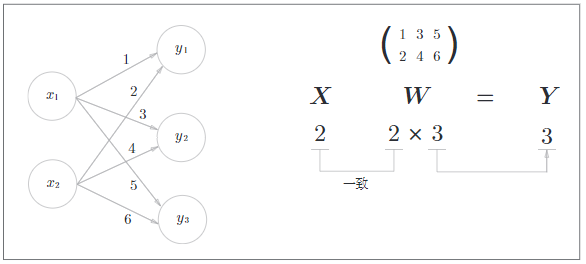``````A = np.array([[1,2]])
B = np.array([[1,3,5],[2,4,6]])
C = np.dot(A,B)
print(C,"\n")
print("A:",A.shape,"* B:",B.shape)
print("C:",C.shape)
``````

``````[[ 5 11 17]]

A: (1, 2) * B: (2, 3)
C: (1, 3)
``````

# 4. 3层数据网络的实现

• 输入层(第0层)有两个神经元，第1个隐藏层(第1层)有三个神经元，第2个隐藏层(第2层)有两个神经元
• 输出层(第3层)有两个神经元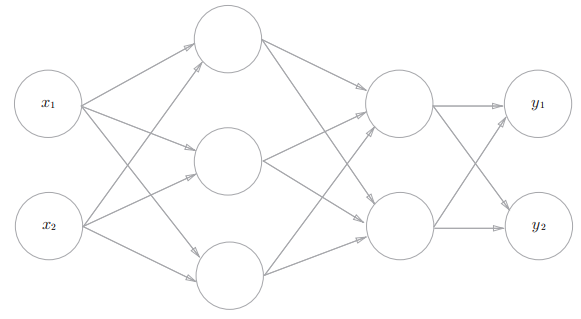## 4.1 符号确认: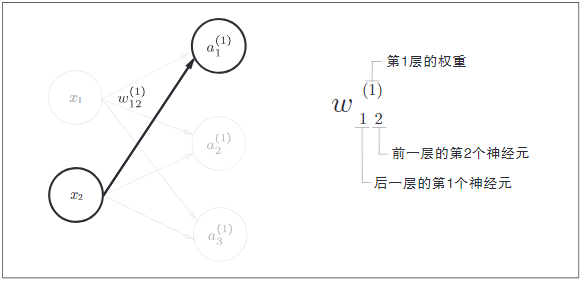## 4.2 第一层神经信号的计算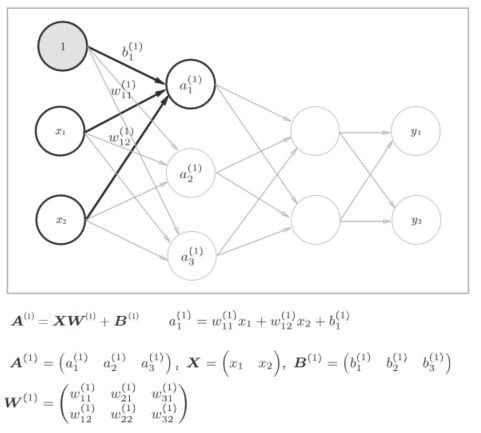• 代码实现:
``````X = np.array([1,0.5])
W1 = np.array([[0.1,0.3,0.5],[0.2,0.4,0.6]])
B1 = np.array([0.1,0.2,0.3])

# 计算加权和
A1 = np.dot(X,W1) + B1

print("A1:",A1,"\n")
print("X:",X.shape,"* W1:",W1.shape, "+ B1:",B1.shape)
print("A1:",A1.shape,"\n")

# sigmoid函数计算
Z1 = sigmoid(A1)
print("Z1:",Z1)
print("Z1:",Z1.shape,"\n")
``````

``````A1: [ 0.3  0.7  1.1]

X: (2,) * W1: (2, 3) + B1: (3,)
A1: (3,)

Z1: [ 0.57444252  0.66818777  0.75026011]
Z1: (3,)
``````

1. 计算隐藏层的加权和(加权信号和偏置的和)，用A1表示：A1 = np.dot(X,W1) + B1
2. 该隐藏层的加权和，被激活函数转换后的信号，用Z1表示:Z1 = sigmoid(A1)

## 4.3 第二层神经信号的计算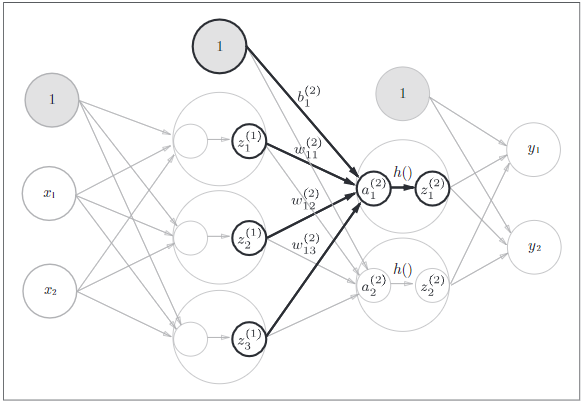• 计算第一层到第二层信号
``````W2 = np.array([[0.1, 0.4], [0.2, 0.5], [0.3, 0.6]])
B2 = np.array([0.1, 0.2])

# 根据上一层的信号，再次计算加权和
A2 = np.dot(Z1, W2) + B2

# sigmoid函数计算
Z2 = sigmoid(A2)
print("Z2:",Z2)
print("Z2:",Z2.shape,"\n")
``````

``````Z2: [ 0.62624937  0.7710107 ]
Z2: (2,)
``````

## 4.4 输出层信号计算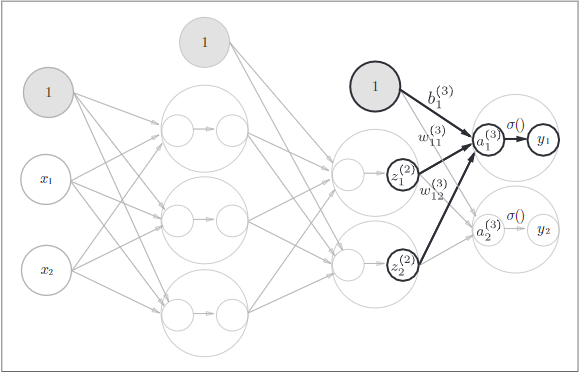``````def identity_function(x):
return x

W3 = np.array([[0.1, 0.3], [0.2, 0.4]])
B3 = np.array([0.1, 0.2])

A3 = np.dot(Z2, W3) + B3

Y = identity_function(A3) # 或者Y = A3

print("Y:",Y)
print("Y:",Y.shape,"\n")
``````

``````Y: [ 0.31682708  0.69627909]
Y: (2,)
``````

## 4.5 代码小结

``````# 初始化权重和偏置，并保存到字典变量network中
def init_network():
network = {}
network["W1"] = np.array([[0.1,0.3,0.5],[0.2,0.4,0.6]])
network['b1'] = np.array([0.1, 0.2, 0.3])
network['W2'] = np.array([[0.1, 0.4], [0.2, 0.5], [0.3, 0.6]])
network['b2'] = np.array([0.1, 0.2])
network['W3'] = np.array([[0.1, 0.3], [0.2, 0.4]])
network['b3'] = np.array([0.1, 0.2])

return network

# 从输入到输出方向的传递处理
def forward(network,x):
W1, W2, W3 = network['W1'], network['W2'], network['W3']
b1, b2, b3 = network['b1'], network['b2'], network['b3']

# 输入 → 第一层
a1 = np.dot(x, W1) + b1
z1 = sigmoid(a1)

# 第一层 → 第二层
a2 = np.dot(z1, W2) + b2
z2 = sigmoid(a2)

# 第二层 → 输出
a3 = np.dot(z2, W3) + b3
y = identity_function(a3)

return y

network = init_network()
x = np.array([1.0,0.5])
y = forward(network,x)
print("Y:",Y)
``````
• `network = init_network()`: 该函数进行权重和偏置的初始化，并将其保存到字典变量network中。
• `x = np.array([1.0,0.5])`：给定输入信号。
• `y = forward(network,x)`：根据输入信号和权重参数，将输入信号转换为输出信号。

# 5. 输出层的设计

• 一般回归问题(预测明天气温)用恒等函数
• 二元分类问题(预测婚否)用sigmoid函数
• 多元分类问题(预测猫狗鼠等)用softmax函数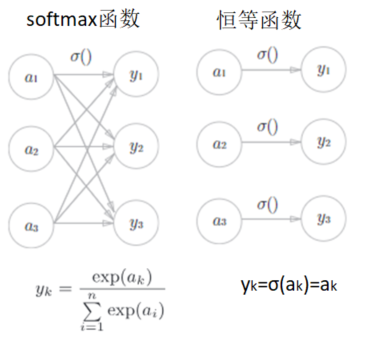1. 使用softmax的输出层的各个神经元，都受到上一层输入信号的影响。(因为分母是所有神经元的总和)
2. 恒等函数不会受到上一层输入信号的影响，输入信号被原封不动的输出。

## 5.1 softmax函数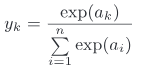``````def softmax(a):
exp_a = np.exp(a)
sum_exp_a = np.sum(exp_a)
y = exp_a / sum_exp_a

return y
a = np.array([0.1, 2.9, 4.0])
print("y:",softmax(a))
``````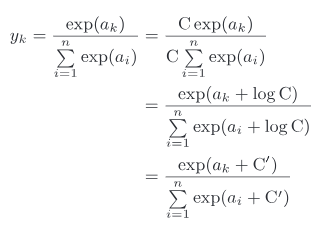``````def softmax(a):
c = np.max(a)
exp_a = np.exp(a - c)
sum_exp_a = np.sum(exp_a)
y = exp_a / sum_exp_a

return y

a = np.array([1010, 1000, 990])
print("y:",softmax(a))
``````

``````def softmax(a):
c = 0
exp_a = np.exp(a - c)
sum_exp_a = np.sum(exp_a)
y = exp_a / sum_exp_a

return y

a = np.array([1010, 1000, 990])
print("y:",softmax(a))
``````

softmax函数的特征

• softmax函数的输出值总和是1，输出总和是1是softmax函数的一个重要性质，所以可以把softmax函数的输出解释为概率
• 即便有了softmax函数，因为指数函数`y=exp(x)`是单调递增函数，各个输入信号的大小关系没有变化，比如输入信号a的最大值是第2个元素，y输出信号的最大值也会是第2个元素。

• 学习：在学习阶段进行模型的学习，即用训练数据自动调整参数的过程。
• 推理：然后，在推理阶段，用学到的模型对未知的数据进行推理分类 在推理阶段一般会省略输出层的softmax函数，在输出层使用softmax函数是因为它和神经网络的学习有关系。

## 5.2 输出层的神经元数量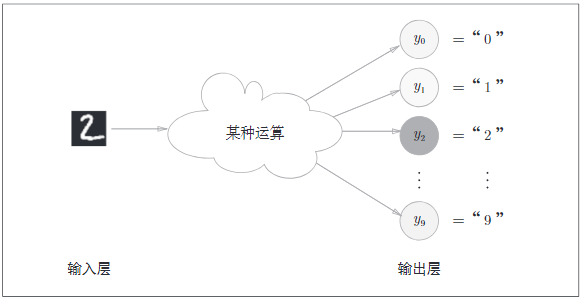# 6. 手写数字识别

MINIST数据集是手写数字的图像集，由0-9数字图像构成，训练图像6万多张，测试图像1万多张，这些图像用来学习和推理，Minist数据集的一般使用方式为:

1. 先用训练图像进行学习
2. 再用学习到的模型，衡量能多大程度上对图像进行正确的分类

MINIST图像数据是28*28像素的灰度图像，各个像素取值在0-255之间，每个图像数据也有对应的1,2,3等标签。

• 读取数据并查看：
``````# coding: utf-8
import sys, os
sys.path.append(os.pardir)  # 为了导入父目录的文件而进行的设定
import numpy as np
from PIL import Image

def img_show(img):
pil_img = Image.fromarray(np.uint8(img))
pil_img.show()

# (训练图像，训练标签),(测试图像，测试标签)
(x_train, t_train), (x_test, t_test) = load_mnist(flatten=True, normalize=False)

# 输出各个数据的形状
print(x_train.shape) # (60000, 784)
print(t_train.shape) # (60000, )
print(x_test.shape) # (10000, 784)
print(t_test.shape) # (10000, )

img = x_train
label = t_train
print("\n",label)  # 5

print(img.shape)  # (784,)
img = img.reshape(28, 28)  # 把图像的形状变为原来的尺寸
print(img.shape)  # (28, 28)

img_show(img)

``````
• 先将数据集分成训练集，以及测试集，分别有60000和10000条记录，每条记录上有784个像素数据
• `5`表示该元素的标签，即正确的识别结果
• 将784个一维像素点，转换为二维数据，并显示为图像,图像显示使用PIL(Python Image Libray)模块

``````(60000, 784)
(60000,)
(10000, 784)
(10000,)

5
(784,)
(28, 28)
``````## 6.1 神经网络的推理处理

• 输入层信号:即一个图像数据，该数据有784个神经元，这个784个神经元来自图像大小28*28 = 784 像素。
• 输出层的10个神经元，这个数字来源于10类别分类。
• 这个神经网络有2个隐藏层，第一个隐藏层有50个神经元，第二个隐藏层有100个神经元。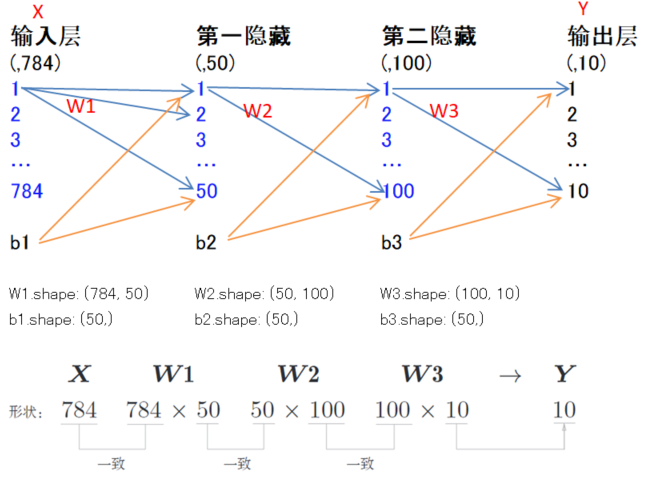``````# coding: utf-8
import sys, os
sys.path.append(os.pardir)  # 为了导入父目录的文件而进行的设定
import numpy as np
import pickle
#from common.functions import sigmoid, softmax

def get_data():
(x_train, t_train), (x_test, t_test) = load_mnist(normalize=True, flatten=True, one_hot_label=False)
return x_test, t_test

def init_network():
with open("./ch03/sample_weight.pkl", 'rb') as f:

return network

def predict(network, x):
W1, W2, W3 = network['W1'], network['W2'], network['W3']
b1, b2, b3 = network['b1'], network['b2'], network['b3']

a1 = np.dot(x, W1) + b1
z1 = sigmoid(a1)
a2 = np.dot(z1, W2) + b2
z2 = sigmoid(a2)
a3 = np.dot(z2, W3) + b3
y = softmax(a3)

return y

x, t = get_data()
network = init_network()

accuracy_cnt = 0
for i in range(len(x)):
y = predict(network, x[i])
p= np.argmax(y) # 获取概率最高的元素的索引
if p == t[i]:
accuracy_cnt += 1

print("Accuracy:" + str(float(accuracy_cnt) / len(x)))
``````
• `get_data()`，获取训练数据和测试数据。
• `init_network()`,读取外部权重和偏置文件。
• `predict()`，根据权重和偏置，使用激活函数和softmax函数，得到输出结果y，y是10个神经元的概率。

## 6.2 批处理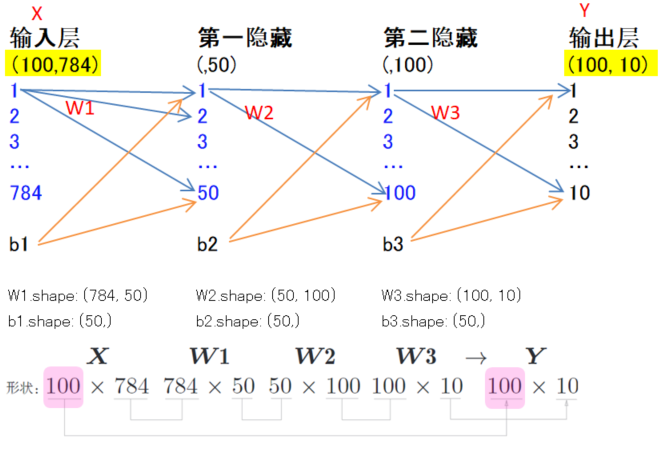• 最后部分修改后代码如下:
``````x, t = get_data()
network = init_network()

batch_size = 100
accuracy_cnt = 0
for i in range(0,len(x),batch_size):
x_batch = x[i:i+batch_size]
print(x_batch.shape)
y_batch = predict(network, x_batch)
print(y_batch.shape)
p = np.argmax(y_batch,axis=1)
accuracy_cnt += np.sum(p == t[i:i+batch_size])

print("Accuracy:" + str(float(accuracy_cnt) / len(x)))
``````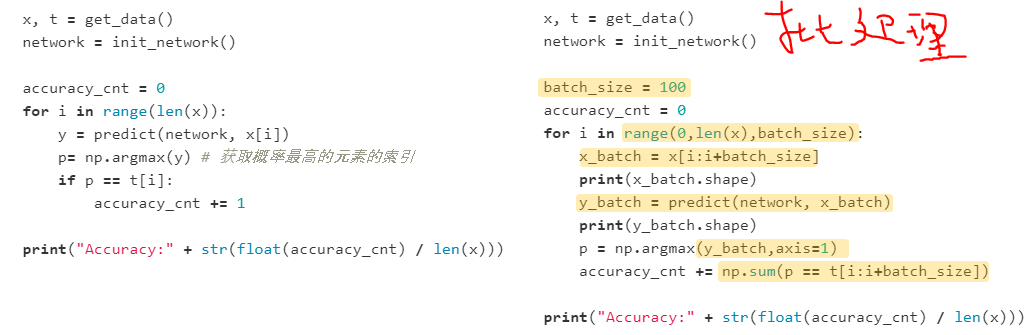1. range()，若range()函数指定为range(start,end)，则会生成一个由start到end-1的整数构成的列表。
2. x_batch = x[i:i+batch_size]，通过这个取得第i个到第i+batch_n个之间的数据。
3. argmax()，获取值最大的元素的索引，这里指定了axis=1，指定了在100*10数组中，沿着第1维方向找到值最大的元素的索引。

# 7. 小结

• 神经网络中使用的是平滑变化的sigmoid函数
• 感知机中使用的是信号急剧变化的阶跃函数

• 神经网络中的激活函数使用平滑变化的sigmoid函数或ReLU函数
• 通过巧妙地使用Numpy多维数组，可以高效实现神经网络
• 机器学习大体分为回归问题(预测年龄)和分类问题(判断性别)
• 关于输出层的激活函数，回归问题中一般使用恒等函数，分类问题中一般用softmax函数(二元分类问题用sigmoid函数，多元分类使用softmax函数)
• 分类问题中，输出层的神经元数量设置为要分类的类别数
• 输入数据的集合称为批，通过以批为单位进行推理处理，能够实现高速运算
• 下面是几个激活函数的示例代码：
``````# sigmoid函数
def sigmoid(x):
return 1 / (1 + np.exp(-x))

# Softmax函数
def softmax(a):
exp_a = np.exp(a)
sum_exp_a = np.sum(exp_a)
y = exp_a / sum_exp_a

return y

# 恒等函数
def identity_function(x):
return x

# ReLU函数，大于0，则输出X，否则输出0
def relu(x):
return np.maximum(0,x)
``````